# Influence of Strip Transverse Temperature Deviation in Hot Rolling Based on Two Dimension Alternating Difference

Influence of Strip Transverse Temperature Deviation in Hot Rolling Based on Two Dimension Alternating Difference

Jian Shao Zengshuai Qiu Bo Huang Wenquan Sun Anrui He

National Engineering Research Center of Advanced Rolling Technology, University of Science and Technology Beijing, China;

Hot Strip Mill of Laiwu Iron and Steel Co., Ltd, Laiwu, China.

Corresponding Author Email:
ustbshao@163.com
Page:
45-50
|
DOI:
ttps://doi.org/10.18280/ijht.330207
N/A
| |
Accepted:
N/A
| | Citation

OPEN ACCESS

Abstract:

According to energy conservation and finite difference method, strip transient temperature model was established with high operation speed and good operation precision, which used discretization mesh in strip cross section, analyzed energy conversion of each control volume, transformed differential equation into difference equation, built various node difference equations, used two dimension alternating difference for equations solution. Based on the transient temperature field model, influence rule of strip transverse temperature was analyzed with different strip thickness, width and slab initial temperature difference. The research results show that strip transverse temperature in finishing stand is direct proportion to strip thickness, width and slab initial temperature difference. The results also provide theoretical support for the transverse temperature difference improvement in hot rolling.

Keywords:

Alternating Difference, Boundary Condition, Energy Conservation, Hot Strip Mill, Transverse Temperature Deviation.

1. Introduction

Temperature is one of the most important factors in metal plastic deformation process. In hot rolling, in order to get qualified performance and rolling stability, single point temperature is more concerned in general, such as finishing temperature control and coiling temperature control, and there is less research on strip transverse temperature deviation, which not only influences transverse microstructure, mechanical and physical properties, but also easily leads to edge wave defects after laminar cooling [1-3]. In order to get the distribution law of the transverse temperature and improve strip quality, research on transient temperature field in the rolling process has positive significance. From the first finishing stand entry side to last finishing stand exit side, strip temperature changes are very complex, including heat conduction between strip and work roll, heat convection between strip surface and cooling water, heat convection between strip surface and ambient air, heat from plastic deformation, friction heat and so on [4-8]. Based on the previous research of rolling temperature field, this paper established a transient temperature field model which considered calculation efficiency and precision, and took into account various kinds of heat transfer phenomenon by using alternating difference method.

Alternating difference method has efficient calculation speed and high precision, which can be used in transient temperature field solution. For a given time step Δt, difference equation is divided into explicit equation and implicit equation. In previous Δt/2 period, explicit scheme is used in x direction and implicit is used in y direction, in later Δt/2 period, implicit scheme is used in x direction and explicit is used in y direction . Compared with explicit difference, alternating difference method has absolute stability, which can allow arbitrary selection time step and spatial step. Compared with implicit difference, alternating difference method has efficient calculation speed, which avoids large linear equations in iterative solution, in each time step, alternating difference solve tridiagonal linear equations using chase method [10-11].

2. Transient Temperature Field Model In Hot Rolling

Usually transient temperature field model inside the solid is composed of heat conduction differential equation based on Fourier law and energy conservation law. Taking differential element from object with side length dx, dy and dz, the heat balance relationship can be expressed as follow:

$c \rho \frac{\partial T}{\partial t}=\lambda\left(\frac{\partial^{2} T}{\partial x^{2}}+\frac{\partial^{2} T}{\partial y^{2}}+\frac{\partial^{2} T}{\partial z^{2}}\right)+q_{\mathrm{in}}$       (1)

Where c is object specific heat, ρ is object density, λ is object thermal conductivity, qin is heat flux of inner heat source. Equation (1) shows the internal relations among the object inside points, by some definite conditions, such as geometric conditions, physical conditions, initial conditions and boundary conditions, transient temperature field can be obtained.

In finishing stand, heat transfer occurs in thickness, width and length direction. Due to less heat transfer in length direction and more concern on strip horizontal temperature distribution, two dimensional alternating difference model for temperature field solution is established. As shown in Fig.1, x is strip width direction, y is strip thickness direction, B is strip width, H is strip thickness, i is node number of x, j is node number of y, strip is divided into many rectangular grids in B×H area, which has NB grids in x direction and NH grids in y direction, also equal interval grid Δx and Δy in both directions. In strip side corresponding to operating side and drive side, heat exchange is occurred between strip and air, qw is heat flow density in operating side, qd is heat flow density in drive side. In strip top and bottom surface, heat exchange is occurred among strip, water and work roll, qt is heat flow density in top surface, qb is heat flow density in bottom surface.

## 1.png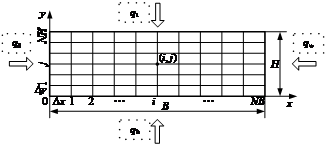Figure 1. Mesh generation of transient temperature field model

According to energy conservation law and finite difference theory, the control volume of each node takes energy analysis, and the differential equations are transformed to difference equations. There are four node types: internal node, edge node in width direction, edge node in thickness direction, corner node, and control volume energy relation of four node types are shown in Fig. 2, the dashed box shaded areas represent the control volume.

## 2.pngFigure 2. Control volume energy relation of four node types

Because of energy relation of each node is not uniform, the difference equations are also different. Taking the internal node for example, in previous Δt/2 period, finite difference equations are established based on energy transfer relation and energy conservation law. In time k to kt, the internal node temperature changes from Tki,j to Tk+1i,j, also, width direction uses implicit difference and thickness direction uses explicit difference.

According to the plate heat conduction principle and taking internal node for example, the heat quantity Q1 of control volume from node (i–1, j–1) to node (i, j) can be expressed as follow:

$Q_{1}=\lambda \Delta y \frac{\Delta t}{2}\left(T_{i-1, j}^{k+\frac{1}{2}}-T_{i, j}^{k+\frac{1}{2}}\right) / \Delta x$   (2)

Also Q2Q3Q4 from other three nodes can be obtained as follows:

$Q_{2}=\lambda \Delta y \frac{\Delta t}{2}\left(T_{i+1, j}^{k+\frac{1}{2}}-T_{i, j}^{k+\frac{1}{2}}\right) / \Delta x$    (3)

$Q_{3}=\lambda \Delta x \frac{\Delta t}{2}\left(T_{i, j-1}^{k}-T_{i, j}^{k}\right) / \Delta y$     (4)

$Q_{4}=\lambda \Delta x \frac{\Delta t}{2}\left(T_{i, j+1}^{k}-T_{i, j}^{k}\right) / \Delta y$    (5)

Inner heat source is as bellow:

$Q_{\text {in }}=q_{\text {in }} \Delta x \Delta y \frac{\Delta t}{2}$     (6)

In previous Δt/2 period, energy increment of node (i, j) control volume is as follow:

$U_{i, j}^{k+\frac{1}{2}}=\rho \Delta x \Delta y\left(T_{i, j}^{k+\frac{1}{2}}-T_{i, j}^{k}\right)$       (7)

According to energy conservation law, energy of node (i, j) control volume is equilibrated:

$Q_{1}+Q_{2}+Q_{3}+Q_{4}+Q_{\mathrm{in}}=U_{i, j}^{k+\frac{1}{2}}$      (8)

Based on Eq.(2)-Eq.(8), two dimensional difference equation of internal nodes in previous Δt/2 period can be expressed as:

$-f_{x} T_{i-1, j}^{k+\frac{1}{2}}+2\left(g+f_{x}\right) T_{i, j}^{k+\frac{1}{2}}-f_{x} T_{i+1, j}^{k+\frac{1}{2}}= f_{y} T_{i, j-1}^{k}+2\left(g-f_{y}\right) T_{i, j}^{k}+f_{y} T_{i, j+1}^{k}+q_{\mathrm{in}}$      (9)

Where $f_{x}=\lambda / \Delta x^{2}, f_{y}=\lambda / \Delta y^{2}, g=\rho c / \Delta t$

Equation (9) can be expressed as matrix:

$a T_{i-1, j}^{k+\frac{1}{2}}+b T_{i, j}^{k+\frac{1}{2}}+c T_{i+1, j}^{k+\frac{1}{2}}=d$    (10)

Where:

$\left\{\begin{array}{l}a=-f_{x} \\ b=2\left(g+f_{x}\right) \\ c=-f_{x} \\ d=f_{y} \cdot T_{i, j-1}^{k}+2\left(g-f_{y}\right) \cdot T_{i, j}^{k}+f_{y} \cdot T_{i, j+1}^{k}+q_{i n}\end{array}\right.$      (11)

Temperature for each j column in previous Δt/2 period can be calculated by using chase method, as shown in Eq.(12).

In later Δt/2 period, implicit scheme is used in thickness direction and explicit is used in width direction, and temperature for each i column is solved. Based on the same method, two dimensional difference equation for all nodes can be achieved.

$\left[\begin{array}{cccccc}b_{0} & c_{0} & & & & \\ a_{1} & b_{1} & c_{1} & & & \\ & \ddots & \ddots & \ddots & & & \\ & & a_{i} & b_{i} & c_{i} & & \\ & & & \ddots & \ddots & \ddots & \\ & & & & a_{N B-1} & b_{N B-1} & c_{N B-1} \\ & & & & & a_{N B} & b_{N B}\end{array}\right]\left[\begin{array}{c}\kappa_{0, j}^{k+\frac{1}{2}} \\ T_{1, j}^{k+\frac{1}{2}} \\ \vdots \\ \\ T_{i, j}^{k+\frac{1}{2}} \\ \vdots & \\ & T_{N B-1, j}^{k+\frac{1}{2}} \\ & T_{N B, j}^{k+\frac{1}{2}}\end{array}\right]=\left[\begin{array}{l}d_{0} \\ d_{1} \\ \vdots \\ d_{i} \\ \vdots \\ d_{N B-1} \\ d_{N B}\end{array}\right]$        (12)

3. Temperature Field Model Definite Conditions

Temperature field calculation is dependent on definite conditions, such as physical conditions and boundary conditions, including thermal conductivity, specific heat capacity, density, internal heat source, heat exchange and so on. It is difficult to get the accurate definite conditions and empirical formula built by experiments is more popular, actual data are also used for correction.

3.1 Physical conditions

Thermal conductivity is a parameter characterizing the strip internal heat conduction ability, which is related to steel chemical composition, density and temperature. Fig. 3 shows the strip thermal conductivity with different carbon content in the range of 200°C to 1200°C. In this paper, strip transverse temperature distribution is the solving object, thermal conductivity along the width direction is different and calculated by interpolation based on Fig. 3.

## 3.png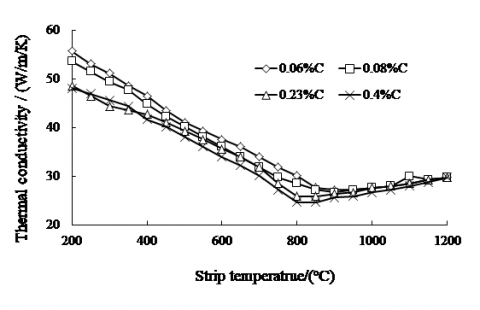Figure 3. Strip thermal conductivity with different carbon content in the range of 200°C to 1200°C

Specific heat capacity is also related to steel chemical composition, density and temperature, Fig. 4 shows the strip specific heat capacity with different carbon content in the range of 200°C to 1200°C.

## 4.png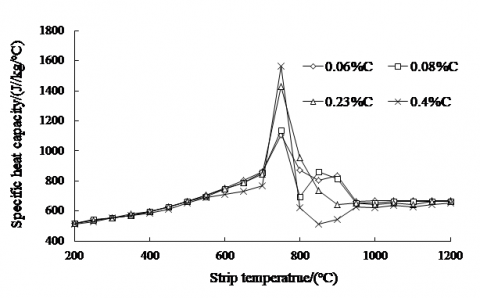Figure 4. Strip specific heat capacity with different carbon content in the range of 200°C to 1200°C

In unit time, calorific value of unit control volume caused by plastic deformation is as follow:

$q_{\mathrm{def}}=2 A \sigma_{\mathrm{s}} \ln \left(\frac{h_{0}}{h_{1}}\right) / \sqrt{3} t_{\mathrm{r}}$   (13)

$t_{\mathrm{r}}=\sqrt{R\left(h_{0}-h_{1}\right)} / v_{\mathrm{r}}$   (14)

Where qdef is calorific value, A is mechanical equivalent of heat, A=0.9, σs is strip average deformation resistance in deformation zone, h0 is strip thickness in mill entry side, h1 is strip thickness in mill exit side, tr is rolling time for deformation zone, R is work roll radius, vr is work roll peripheral speed.

The sum of friction power and deformation power is equal to rolling power:

$2 l_{\mathrm{c}} B \cdot q_{\mathrm{fic}} / \zeta+l_{\mathrm{c}} B \cdot q_{\mathrm{def}} / A=P$  (15)

Where qfric is friction heat in unit area and unit time, ζ is friction heat ratio coefficient absorbed by strip, lc is contact arc length in deformation zone, P is total rolling power. Because the total rolling power is known, when the deformation power is calculated, friction heat in unit area and unit time is achieved

3.2 Boundary conditions

The main heat exchange of strip under air cooling condition is radiation heat transfer and convection heat transfer, heat transfer coefficient can be expressed as:

$h_{\mathrm{a}}=h_{\mathrm{rad}}+h_{\mathrm{conv}}$    (16)

Where, hrad is radiation heat transfer coefficient, hconv is convection heat transfer coefficient.

In stand, radiation heat transfer occurs between strip and air, hrad can be calculated as follow:

$h_{\mathrm{rad}}=\frac{k_{\mathrm{rad}} \varepsilon_{\mathrm{rad}}\left[\left(\frac{273+T_{\mathrm{suf}}}{100}\right)^{4}-\left(\frac{273+T_{\mathrm{env}}}{100}\right)^{4}\right] \times m^{2}}{T_{\mathrm{suf}}-T_{\mathrm{env}}}$     (17)

εrad changed with strip temperature and can be expressed as:

$\varepsilon_{\mathrm{rad}}=\frac{T+273}{1000}\left[0.125 \times \frac{T+273}{1000}-0.38\right]+1.1$        (18)

The convective heat transfer coefficient between the strip and the air is relevant to strip speed vs:

$h_{\mathrm{conv}}=\left\{\begin{array}{ll}5.6+4 v_{\mathrm{s}} & v_{\mathrm{s}}<5 \\ 7.2953 v_{\mathrm{s}}^{0.78} & v_{\mathrm{s}} \geq 5\end{array}\right.$      (19)

Strip surface temperature is much higher than cooling water, and heat exchange coefficient is relevant to cooling water injection mode, water temperature, water quantity, strip surface temperature, surface shape and so on. In hot rolling, strip temperature in stand is usually more than 900°C and the heat transfer is film boiling, heat transfer coefficient can use regression formula as below:

$h_{\mathrm{w}}=1.163 \times 107.2 \mathrm{w}^{0.663} \times 10^{-0.00147T \mathrm{suf}}$   (20)

Where w is water flow density. The heat transfer calculation between strip and work roll need the heat transfer coefficient under different conditions, and empirical formula or semi-empirical formula is popular. Heat transfer coefficient between strip and work roll is relevant to oxide scale thickness, lubricating medium, strip temperature and so on, the calculation formula is as follow:

$h_{\mathrm{s}}=\frac{\left(\lambda_{\mathrm{r}} \times \lambda_{\mathrm{s}}\right) \times\left(2.1 e^{-9} p_{\mathrm{m}}\right)^{1.7}}{\left(\lambda_{\mathrm{r}}+\lambda_{\mathrm{s}}\right) 35 e^{-6}}$    (21)

Where, hs is heat transfer coefficient between strip and work roll, λr is work roll thermal conductivity, λs is strip thermal conductivity, pm is unit rolling force in deformation area.

4. Calculation Flow of Strip Transient Temperature Field

Definite conditions of strip transient temperature model based on two dimension alternating difference are given in section 3. Taking 7 stands hot strip mill for example, by getting parameters of the equipment and heat exchange conditions in stands, strip transient temperature is achieved.

In order to ensure the universality of calculating program, finishing stand is divided into different heat exchange area in strip temperature calculation according to equipment and process characteristic of most hot strip mills. The calculation flow chart is shown in Fig. 5.

## 5.pngFigure 5. Calculation flow chart of strip transient temperature field

5. Influence Rule of Strip Transverse Temperature Under Different Rolling Parameters

5.1 Variation of strip transverse temperature in stands

According to the actual data from production line, optimized strip transient temperature field calculation model can be used in strip temperature simulation. Based on actual conditions, slab temperature before stand 1 is defined, the initial temperature of internal point in the middle width is 1130°C, the maximum temperature difference between internal point and surface is 50°C, the maximum temperature difference of internal point is 70°C, and the maximum temperature difference of internal point is 35°C.

Based on above model, strip transient temperature of each stand is achieved. Because the strip temperature distribution law of each stand is more similar, temperature of stand 7 exit side is shown in Fig. 6 and the characteristics of strip temperature are as follows:

## 6.png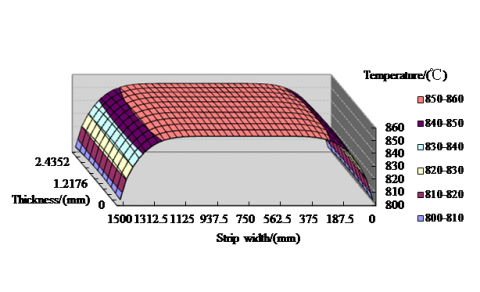Figure 6. Strip transient temperature field of stand 7 exit side

1) From stand 1 to stand 7, the maximum temperature difference in strip thickness direction increases at first then decreases and changes greatly. For example, in width center, initial temperature difference in strip thickness direction is 50°C, in stand 1 exit side, the temperature difference is 110°C, and in stand 7 exit side, the temperature difference is 2°C.

2) From stand 1 to stand 7, the maximum temperature difference in strip width direction increases at first then decreases and changes small. For example, in strip surface, initial temperature difference in strip width direction is 35°C, in stand 1 exit side, the temperature difference is 48°C, and in stand 7 exit side, the temperature difference is 32°C. In addition, compared with initial slab before stand 1, temperature drop zone length in each stand increases about 50mm.

5.2 Strip transverse temperature affected by thickness

In simulation model, strip width of product is 1250mm, thickness of product is respectively 1.8mm, 3.0mm, 5.0mm, according to actual rolling data, and same initial temperature distribution of slab, strip section temperature distribution is achieved by transient temperature field model. As shown in Fig.7, the thickness is small, the maximum transverse temperature difference and temperature drop zone length (5°C deviation compared with middle point) are also small, when thickness is 1.8mm, the transverse temperature difference is 22.3°C and temperature drop zone length is 100mm, when thickness is 5.0mm, the transverse temperature difference is 41.4°C and temperature drop zone length is 160mm.

## 7.png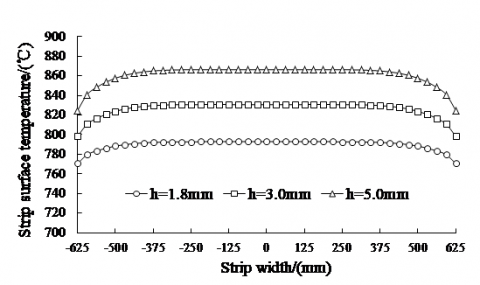Figure 7. Strip transverse temperature in stand 7 exit side affected by different thickness

5.3 Strip transverse temperature affected by width

In simulation model, h is 3.0mm, B is respectively 1020mm, 1250mm, 1500mm, and other conditions are the same as above. As shown is in Fig. 8, width is small, the maximum transverse temperature difference and temperature drop zone length (5°C deviation compared with middle point) in exit side of stand 7 are also small, when width is 1020mm, the transverse temperature difference is 29.6°C and temperature drop zone length is 120mm, when width is 1500mm, the transverse temperature difference is 49°C and temperature drop zone length is 220mm.

## 8.png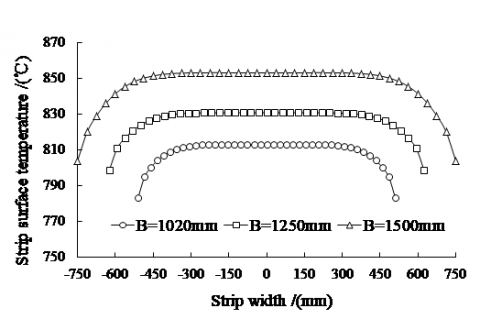Figure 8. Strip transverse temperature in stand 7 exit side affected by different width

5.4. Strip transverse temperature affected by slab initial temperature

In simulation model, slab maximum transverse temperature difference ΔT is respectively 0°C, 35°C, 70°C, h is 3.0mm, B is respectively 1250mm, and other conditions are the same as above. As shown is in Fig. 9, ΔT is small, the maximum transverse temperature difference and temperature drop zone length (5 °C deviation compared with middle point) in exit side of stand 7 are also small, when ΔT is 0°C, the transverse temperature difference is 12.4°C and temperature drop zone length is 25mm, when ΔT is 70°C, the transverse temperature difference is 32.3°C and temperature drop zone length is 150mm.

## 9.png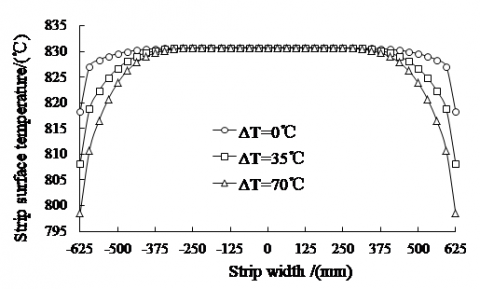Figure 9. Strip transverse temperature in stand 7 exit side affected by slab initial temperature

6. Summary

(1) Strip transient temperature model is established based on energy conservation and finite difference method, and two dimension alternating difference is used for equations solution, which can get high operation speed and good operation precision and be used for online application.

(2) Influence rule of strip transverse temperature under different rolling parameters is studied. The results show that from stand 1 to stand 7 the maximum temperature difference in strip thickness direction increases at first then decreases and changes greatly, the maximum temperature difference in strip width direction increases at first then decreases and changes small. Strip transverse temperature in finishing stand is direct proportion to strip thickness, width and slab initial temperature difference.

Acknowledgement

This work was supported in part by National Natural Science Funds of China (51404021), Fundamental Research Funds for the Central Universities (FRF-TP-14-103A2) and Beijing Municipal Natural Science Foundation (3154035).

References

1. H. B. Li, J. Zhang and Y. M. Zhang, Analysis of work roll thermal behavior for 1450mm hot strip mill with genetic algorithm, Journal of Theoretical and Applied Information Technology, vol. 43, pp. 176-180, 2012.

2. R. Zahradník, J. Hrabovský and M. Raudenský, Study of the work roll cooling in hot rolling process with regard on service life, Metallurgia Italiana, vol. 106, pp. 21-28, 2014.

3. K. Komori and M. Suzuki, Simulation of deformation and temperature in press roll piercing, Journal of Materials Processing Technology, vol. 169, pp. 249- 257, 2005.

4. F. D. Fischer, W. E. Schreiner and E. A. Werner, The temperature and stress fields developing in rolls during hot rolling, Journal of Materials Processing Technology, vol. 150, pp. 263-269, 2004.

5. J. Chen and J. G. Wang, Improved hybrid implicitexplicit finite difference time-domain method with higher accuracy and user defined stability condition, International Journal of Electronics and Communications, vol. 65, pp. 307-317, 2011.

6. K. Komori and K. Katsuhiko, Simulation of deformation and temperature in multi-pass H-shape rolling, Journal of Materials Processing Technology, vol. 105, pp. 24-31, 2000.

7. H. L. Ding, T. Y. Wang and L. Yang, FEM modeling of dynamical recrystallization during multi-pass hot rolling of AM50 alloy and experimental verification, Transactions of Nonferrous Metals Society of China, vol. 23, pp. 2678-2685, 2013.

8. K. X. Peng, Calculation and analysis of temperature distribution in hot rolling strip, Indonesian Journal of Electrical Engineering, vol. 11, pp. 3945-3956, 2013.

9. J. Shao, W. Q. Sun and A. R. He, Work roll temperature prediction model in hot strip mill based on two dimensional alternating difference method, Advanced Materials Research, vol. 652, pp. 2057-2061, 2013.

10. C. G. Sun, H. D. Park and S. M. Hwang, Prediction of three dimensional strip temperatures through the entire finishing mill in hot strip rolling by finite element method, Iron and Steel Institute of Japan International, vol. 42, pp. 629-635, 2002.

11. C. Devadas, D. Baragar and G. Ruddle, Thermal and metallurgical state of steel strip during hot rolling, Metallurgical Transactions, vol. 22, pp. 307-317, 1991.